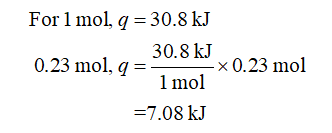# 1)  The molar enthalpy of vaporization of benzene at its boiling point (353K) i3 30.8 kJ mol-1. How much heat is needed to convert 18 g of benzene from liquid into vapors at 353 K if external pressure is 1.0 atm. What work will be done during this process?

Question
38 views
1. 1)  The molar enthalpy of vaporization of benzene at its boiling point (353K) i3 30.8 kJ mol-1. How much heat is needed to convert 18 g of benzene from liquid into vapors at 353 K if external pressure is 1.0 atm. What work will be done during this process?

check_circle

Step 1

The number of moles of benzene is calculated as shown below where n, m, and mm are the number of moles, mass, and molar mass of the benzene respectively. The values for m and mm are substituted.

Step 2

According to the given data, 30.8 kJ of heat is required to vaporize the 1 mol or 78.11 g of liquid benzene. So, the energy required (q) to vaporize the 0.23 mol of benzene is calculated as shown below. The energy required (q) to vaporize the 0.23 mol of benzene is 7.08 kJ.Step 3

The heat of 7.08 kJ is used by the system to increase the internal energy of the system and do some mechanical work due...

### Want to see the full answer?

See Solution

#### Want to see this answer and more?

Solutions are written by subject experts who are available 24/7. Questions are typically answered within 1 hour.*

See Solution
*Response times may vary by subject and question.
Tagged in

### Chemical Thermodynamics